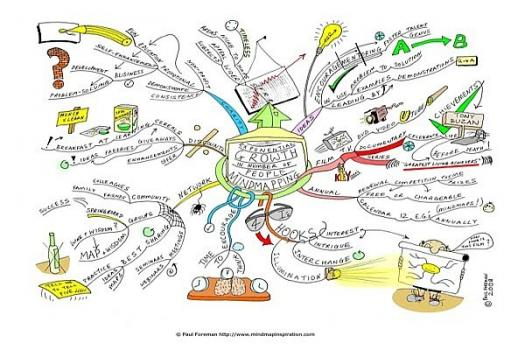# What Do You Know About Discrete Geometry?

10 Questions | Total Attempts: 11SettingsDiscrete geometry and combinatorial geometry are branches of geometry that study combinatorial properties and constructive methods of discrete geometric objects. Most questions in discrete geometry involve finite or discrete sets of basic geometric objects, such as points, lines, planes, circles, spheres, polygons, and so forth.

• 1.
Discrete geometry is branch of ......?
• A.

Geometry

• B.

Algebra

• C.

Vector spaces

• D.

Number theory

• 2.
Discrete geometry is a topic or subject under ......?
• A.

Music

• B.

Mathematics

• C.

Language

• D.

Philosophy

• 3.
The modern discrete geometry has its origin in the ....?
• A.

19th century

• B.

20th century

• C.

21st century

• D.

18th century

• 4.
The following are objects in discrete geometry, expect one.
• A.

Polytopes

• B.

Polyhedra

• C.

Abstract polytopes

• D.

Linear algebra

• 5.
How many topics do we have in discrete geometry?
• A.

Two

• B.

Three

• C.

One

• D.

Five

• 6.
There are how many aspects of polytopes studied in discrete geometry?
• A.

5

• B.

2

• C.

4

• D.

3

• 7.
One of the following is one of the people that laid foundations of discrete geometry.
• A.

H.S.M Coxeter

• B.

Isaac Newton

• C.

James McCaffrey

• D.

Albert Einstein

• 8.
Discrete geometry can be taught in only ..... class?
• A.

History

• B.

Music

• C.

Mathematics

• D.

Philosophy

• 9.
Most questions in discrete geometry involve finite or discrete sets of basic geometric objects except ....
• A.

Planes

• B.

Lines

• C.

Points

• D.

Calculator

• 10.
Discrete geometry is closely related to subjects like the following except ......
• A.

Toric Geometry

• B.

Linear algebra

• C.

Finite geometry

• D.

Digital geometryBack to top